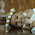For those who are confused with question, we will go to a simple example our given number is 15,
so its positive representation is 1 to 15 and number of times 1 present is 8   i.e 1,10,11,12,13,14,15
now I hope you have understood what we want to achieve

so now we will write a function which returns the number count as result.
we will pass all numbers in a for loop and we will take the mod of that number with 10  inside a while loop
and the result is 1 we will add the count by 1  i.e 1 is present there. and divide that number by 10 for exiting the while loop and finding the count of 1's in integers value more than 10.

if you are checking the mod result equal to 2 you can find out the count of 2, if its 3 count of 3 like wise

public static int Solution(int n)
{
int nums = 0;
int digits = 0;
int count = 0;
for (int i = 1; i <= n; i++)
{
nums = i;
while (nums != 0)
{
digits = nums % 10;
if (digits == 1)
{
count++;
}
nums = nums / 10;
}
}
return count;

}

static void Main(string[] args)
{
Console.WriteLine("Enter the number");
int num =Convert.ToInt32(Console.ReadLine());

int val = Solution(num);

Console.WriteLine("Count of 1s is :" + val);

}

/* androidsharp */

#### 2 comments:

1.Let the language work for you:
-----------------------------------------------
static void Main(string[] args)
{
int target = 15;
int count = 1;
for (int i = 2; i <= target; i++)
{
count += i.ToString().Count(c => c == '1');
}

Console.Write(count);
}
-------------------------------------

2.using lambda expressions :)